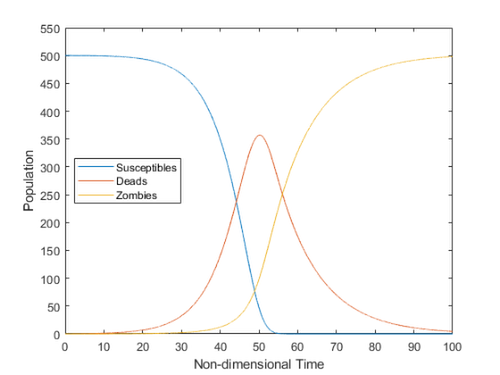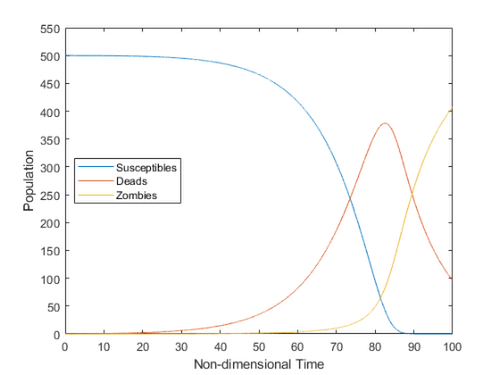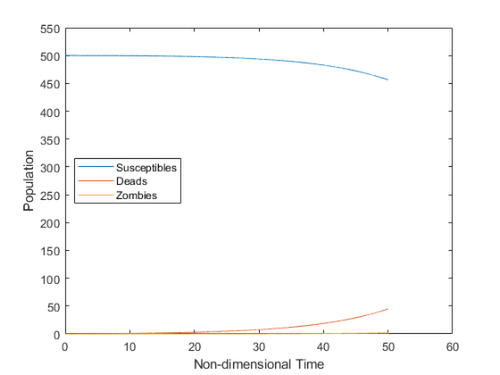## File Exchange Pick of the WeekOur best user submissions

### This is machine translation

Mouseover text to see original. Click the button below to return to the Original version of the page.

# Zombie Outbreak1

Posted by Sean de Wolski,

Sean‘s pick this week is Mathematical modelling of an outbreak of zombie infection by E. Cheynet.

In honor of Halloween, E. has provided us with a way to simulate zombie outbreaks! Do you have what it takes to save humanity?

Npop = 500; % population
alpha = 7.5e-3 ; %  "zombie destruction" rate.
beta = 5e-3; % "zombie creation" rate.
zeta = 9.2e-2; % zombie resurrection rate
delta = 1.2e-4; % susceptibles death rate
tmax = 100; % max duration of the simulation (non-dimensional)
dt = 0.005; % time step

[SRZ, t] = zombies(Npop, alpha, beta, zeta, delta, tmax, dt, 0);
plotPopulations(t, SRZ, Npop)
snapnowHmm, what if we work on our sharp shooter skills and eat healthy?

alpha = 1e-2; % Go to the range
delta = 9e-5; % Add broccoli
[SRZ, t] = zombies(Npop, alpha, beta, zeta, delta, tmax, dt, 0);
plotPopulations(t, SRZ, Npop)
snapnowThat only delays the inevitable; skip the broccoli, we need to execute a mass extermination of zombies. The erad function gives us the ability to raid them.

delta = 1.2e-4; % Back to pizza
k = 0.65;
Nraid = 4;
[SRZ, t] = erad(Npop, alpha, beta, zeta, delta, k, Nraid, tmax, dt,0);
plotPopulations(t, SRZ, Npop)
Zombies have been eradicated (until now...).Unfortunately, the model doesn’t seem to account for new humans being born, so I think any simulation eventually ends with us all dead… Have a great weekend!

Give it a try and let us know what you think here or leave a comment for E.

function plotPopulations(t, SRZ, Npop)
figure
plot(t, SRZ);
ylim([0, 1.1*Npop])
ylabel('Population')
xlabel('Non-dimensional Time')
end


Get the MATLAB code

Published with MATLAB® R2018b